# Cumulative functions

Cumulative formulas allow you to calculate the average, max, min, or sum of your data over time. Although we usually talk about cumulative formulas over time, you can use them over any other sequential data. Each of the cumulative formulas accepts a measure and one or more optional grouping by an attribute (like region or department):

`formula (measure, [attribute, attribute, ...])`

Only the measure value is required. If you supply both a measure and attributes, the formula returns the aggregate of the measure accumulated by the attribute(s) in the order specified. You should experiment with only a measure and then with an attribute to see which output best meets your use case. Note that the cumulative formulas reset their results for different values of any attribute in your search that is not part of your cumulative formula.

The cumulative formulas are:

Function Description

`cumulative_average`

Takes a measure and one or more attributes. Returns the average of the measure, accumulated by the attribute(s) in the order specified. For example: `cumulative_average (revenue, order date, state)`

`cumulative_max`

Takes a measure and one or more attributes. Returns the maximum of the measure, accumulated by the attribute(s) in the order specified. For example: `cumulative_max (revenue, state)`

`cumulative_min`

Takes a measure and one or more attributes. Returns the minimum of the measure, accumulated by the attribute(s) in the order specified. or example: `cumulative_min (revenue, campaign)`

`cumulative_sum`

Takes a measure and one or more attributes. Returns the sum of the measure, accumulated by the attribute(s) in the order specified. For example: `cumulative_sum (revenue, order date)`

You may have attributes in your search that are not part of the cumulative formula. The cumulative formula resets its results for different values of any attribute that is not part of your cumulative formula. For example, with a cumulative formula of `cumulative_sum (sales, date)`, if your search includes the attribute `item type`, the cumulative sum formula aggregates the sales by date separately for different item types. For example: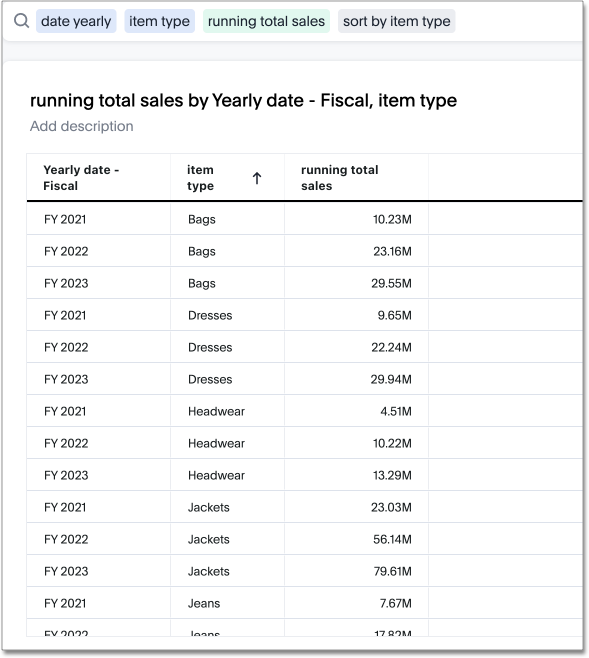## Calculate a cumulative sum

This example demonstrates using the cumulative_sum formula, also known as a running total. To use the cumulative function in a search:

1. Start a new search.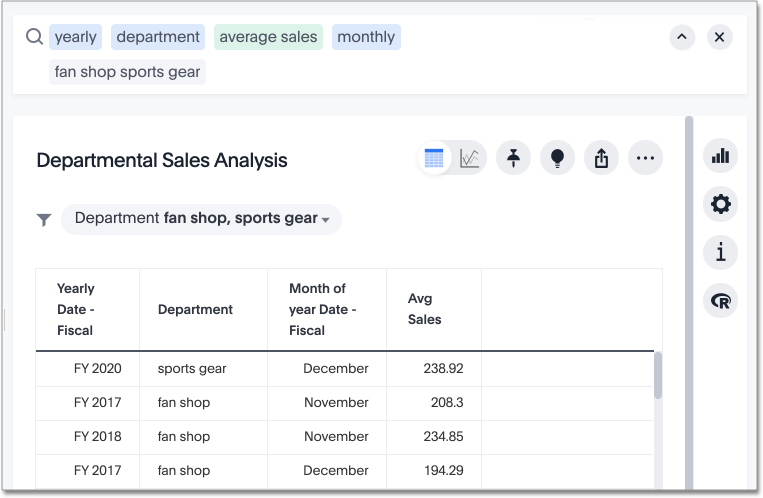2. Open the Data panel from the upper-left corner if it is not open, and select the + icon next to Formulas. If the new Answer experience is off in your environment, select the more options icon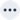in the upper-right side of the table, and select Add formula.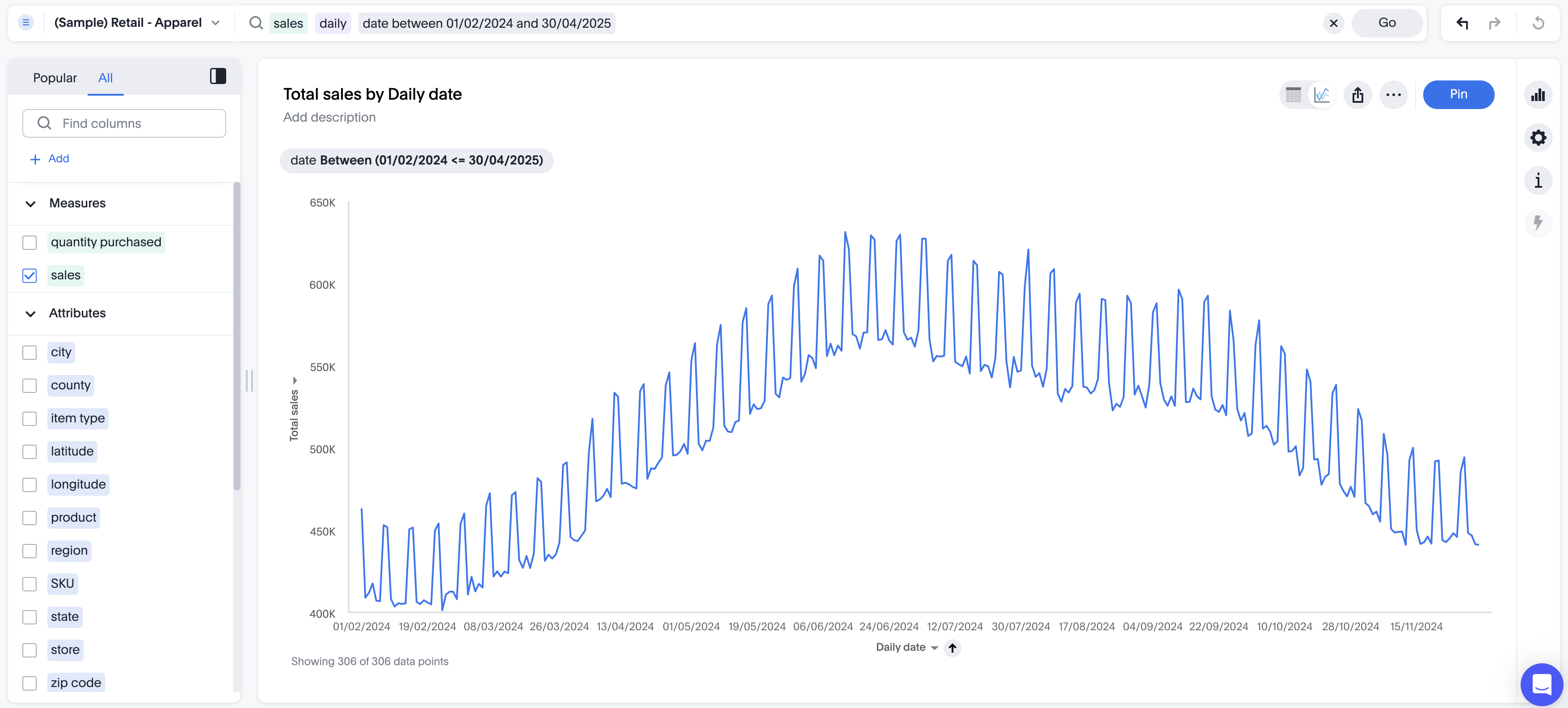3. Enter the cumulative_sum formula, providing a measure and one or more attributes.

This example returns the sum of yearly income, accumulated by the date.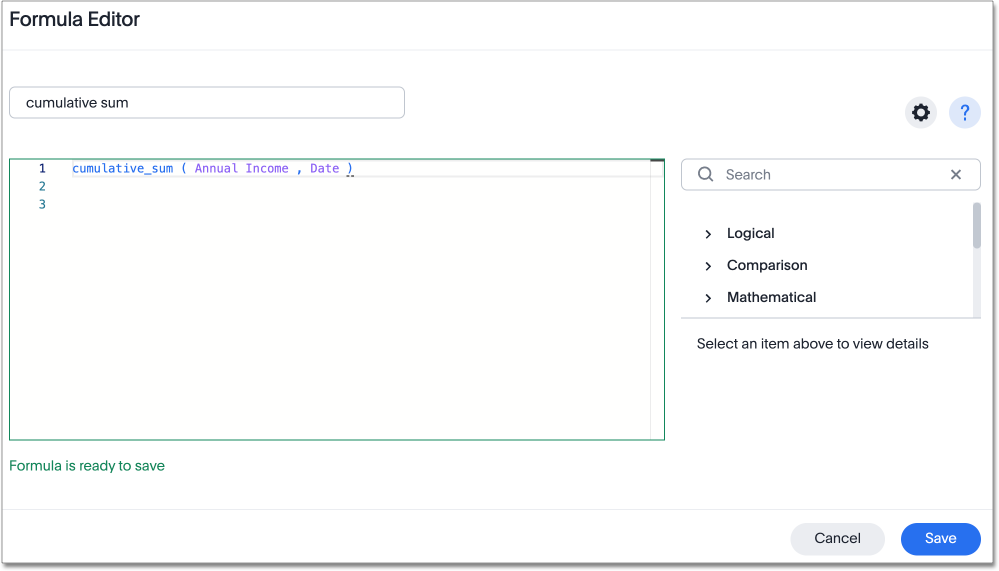4. Name the formula by selecting its title and typing a new name.

5. Select Save.

The formula will appear in the search bar and in the table as its own column.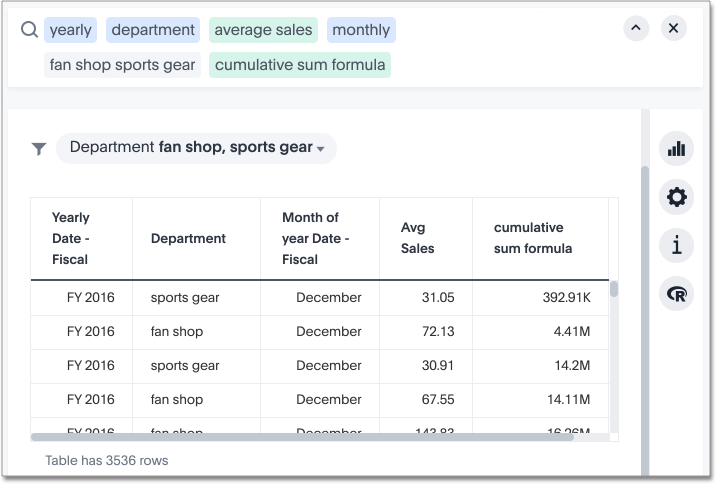A table summary displaying the cumulative sum within the entire table will appear at the bottom. You can select it to toggle between different aggregations.

## Calculate a cumulative sum of a unique count

ThoughtSpot doesn’t have a purpose-built function for calculating a cumulative sum, or other cumulative formula, for a unique count. However, for many use cases, you can use a `group_aggregate` to facilitate this type of count. Adding a `group_aggregate` to your formula allows you to pass the aggregate function (in this case, `unique count`) in the windowing function (in this case, `cumulative_sum`).

See the following example for the general formula for a cumulative sum of a unique count. In this example, we calculate the cumulative sum of unique customer names, using `monthly date` as our aggregation bucket.

``cumulative_sum (group_aggregate (unique count (Customer Name ),query_groups (),query_filters ()),start_of_month (Date) )``

The result looks something like this: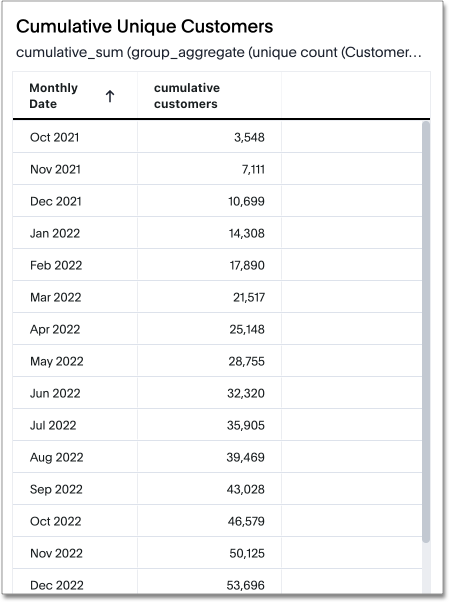You can use this syntax on any cumulative formula: average, maximum, minimum, and sum.

 This syntax produces a unique count for each individual result, such as Oct 2021. However, it doesn’t produce a unique count across results. If a customer name appears in both October 2021 and November 2021, for example, that customer would be counted in both those months.